# Equations of State (EOS)

by Sylvia-Monique Thomas, University of Nevada Las Vegas

# Introduction

What is an Equation of State (EoS)?

An EoS is the relation between functions of state, such as temperature (T), pressure (P), volume (V), internal energy or specific heat. It characterizes the state of matter of a material under a given set of physical conditions. EoSs are used to describe gases, fluids, fluid mixtures and solids. In mineral physics an EoS is used to determine mineral composition at depth (i.e., P and T) and depicts how the volume or equivalently density of a material vary as a function of depth, i.e., as a function of P and T. It reflects atomic structure, chemical bonding and stability of a material.

# Types of Equations of State

The simplest equation of state is the one for ideal gases:

PV = nRT

where n is the number of moles and R is a constant, the gas constant R = 8.31451 J K-1 mol-1. For a volume of ideal gas (V) experiencing an external pressure (P), there will be a related increase in temperature of the gas given by PV / R.

However, at high pressure and at low temperature, real gases deviate from the ideal gas law and the equation needs to be modified.

The EoS for solids and liquids also needs to be modified, more complex EoSs are necessary and various equations have been proposed, which will be discussed below.

For most solids and liquids, the effect of pressure is much greater than the effect of temperature, over the range of temperatures and pressures relevant to the Earth. Therefore it is easier to consider initially an isothermal EOS and then introduce a thermal expansion coefficient as temperature correction.

All EoS are based on assumptions. They are tested by their ability to reproduce the experimental data. Here we will focus on the most commonly used equations. We will start by examining the effect of pressure, i.e. the isothermal EoS.

Isothermal Equation of State

The simplest isothermal EoS for a solid is the bulk modulus or incompressibility, K:

K = -V (∂P/∂V)

where P/V is the partial derivative of pressure with respect to volume. The bulk modulus is a measure of the ability of a material to withstand changes in volume when under uniform compression.

This equation is only valid for a constant K or at P < K, because with increasing P a material's bulk modulus increases, the material becomes stiffer, hence a new formulation is required for a linearly increasing K:

K = K0 + K0' P

where K0 is the bulk modulus at room pressure and K0'=(∂K/∂P)P=0 is the pressure derivative of K, which is assumed to be pressure-dependent.

After integration this leads to the Murnaghan EoS.

1) Murnaghan - 1937

The Murnaghan EoS can be derived from the assumption that the bulk modulus varies linearly with pressure, which results in:

V = V0 (1+K'P/ K0)

This EoS is widely used and known to reproduce both, P-V data and the correct values of the room pressure bulk modulus for compressions up to about 10%.

2) Birch-Murnaghan - 1947

The Birch-Murnaghan EoS (BMEOS) is the most widely used EoS by mineralogists. It's based on the Eulerian strain (fE):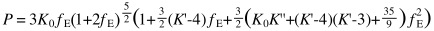In the 2nd order BMEOS K0' is set to a fixed value of 4. The 3rd order BMEOS is used at very high pressures where DK/DP varies significantly with pressure. It is a 3-parameter EoS (V0, K0 and K'') with fE2 = 0 and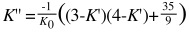In the 4th order BMEOS K'' varies as function of pressure, and can be used for extremely condensed materials.

3) Natural strain EoS / Poirier and Tarantola – 1998

It's a logarithmic EoS, based on the 'Hencky' measure of linear strain or 'natural' strain (fN).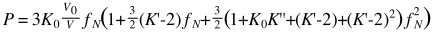In contrast to the 2nd order BMEOS here K' = 2 at second order. In the 3rd order EoS K'' is usually much larger than K'' in the 3rd oder BMEOS: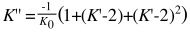.

4) Vinet EoS / 'Universal EoS' – 1986

It is used for solids at very high pressure and and is based on the Vinet strain (fV). It corrects finite-strain EoS, such as the BMEOS, by incorporating volume variation of solids under high-pressure conditions.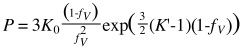with K' = 1 and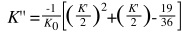.

Thermal Equation of State

The simplest isobaric EOS for a solid is just the definition for the thermal expansion coefficient, a.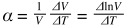A thermal EoS describes a material's reaction to temperature changes. When a material is heated, the thermal pressure in the system changes and results in thermal expansion. One example of EoS used for high-temperature (up to 1000 K) and high-pressure data is the high-temperature BMEOS.

1) High-temperature Birch-Murnaghan EoS

Thermal expansion coefficient and bulk modulus are assumed to vary linearly with temperature,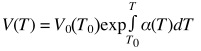,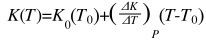where T0 is the reference temperature (298 K).

# Techniques

1) Experimental Determination of an Equation of State

P-V-T data are experimentally determined utilizing a variety of shock compression, multi-anvil or diamond anvil cell experiments in combination with diffraction techniques.

2) Data Analysis: Least-squares fitting

EoS parameters are best estimated using least-squares fitting with pressure usually chosen as the dependent variable, P = f(V,T). The choices of which parameters to refine and which parameters to fix are critical for final fit values and their interpretation. Details about refinement strategy can be found in .

3) Data Analysis: Visual quality assessment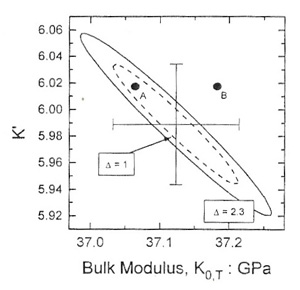Confidence ellipses. Confidence ellipses indicate the region, which by high chance (percentage) contains the 'true values' of the refined parameters K0 and K'. In addition, the slope is used to infer the correlation of the calculated variables. Here, the negative slope indicates the strong negative correlation of K0 and K'.

• Confidence ellipses

Confidence ellipses are visual quality assessment tools. They visualize the correlation coefficient between independently determined values for K0 and K'. The correlation coefficient indicates if two parameters, such as in this case K0 and K', are independent or dependent of one another. The coefficient has a value between -100% and +100% (-1 to +1). If the value is 0, the parameters are independent; if it is below or above zero, the parameters depend on each other partially; if the value is positive a simultaneous increase of both parameters will result in a similar fit; if the value is negative, then increasing one parameter and decreasing the other will lead to a similar fit; if the value is -1 or +1 the parameters completely depend on one another. Details about the calculation of confidence ellipses can be found in .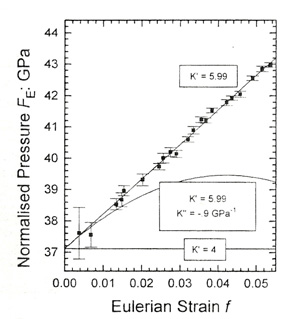F-f plot based on the Birch-Murnaghan EoS. The plot shows results for different K' and K'' values to illustrate the capability of an F-f plot to indicate which EoS is necessary to fit the data.

• F-f-plot

The F-f plot is another visual quality assessment tool for finite strain EoS, where fE (abscissa) is plotted against FE(y-axis), a 'normalised' stress. Based on the F-f plot one can determine the compressional behavior of a material.

a) Data points plot on a horizontal line of constant F; K' = 4; data can be fitted with a 2nd order BMEOS.

b) Data points plot on an inclined straight line; slope is equal to 3K0(K'-4)/2; data can be fitted with a 3rd order BMEOS.

c) Data points plot on a parabolic curve; K'' differs from implied value; f2 ≠ 0.

• Sources of error
1. Uncertainties in volume measurement (incl. V0 errors)
2. Uncertainties in pressure determination
3. Small data-set (low number of data points)

# Guest Lecturer

"Equations of State" Prof. Ross J. Angel

Part 1 (35 minutes) (MP4 Video 42.3MB Jul12 21)

Part 2 (60 minutes) (MP4 Video 70.5MB Jul12 21)

# Literature

 Anderson, O.L. (1995) Equations of State of Solids for Geophysics and Ceramic Science. Oxford University Press, Oxford, 405 pp.

 Angel, R.J. (2000) Equations of state. In: R.M. Hazen and R.T. Downs (Eds.) High-temperature and high-pressure crystal chemistry, Reviews in Mineralogy and Geochemistry, 41, 35-60.

 Duffy, T.S. and Wang, Y. (1998) Pressure-volume-temperature equations of state. In: R.J. Hemley (Ed.) Ultrahigh-pressure mineralogy: physics and chemistry of the Earth's deep interior, Reviews in Mineralogy, 37, 425-457.

 Poirier, J.P. (2000) Introduction to the Physics of the Earth Interior. Second edition, Cambridge University Press, Cambridge, 312 pp.

Technical Papers:

 Murnaghan, F.D. (1937) Finite deformations of an elastic solid. American Journal of Mathematics, 59, 235–260.

 Birch, F. (1947), Finite elastic strain of cubic crystals, Physical Review, 71, 809-824.

 Stacey, F. D., Brennan, B. J. and Irvine, R. D., 1981. Finite strain theories and comparisons with seismological data. Geophysical Surveys, 4, 189-232.

 Jeanloz, R. (1988) Universal Equation of State. Physical Review B, 38, 805-807.

# Problem Set

EoS Homework (Acrobat (PDF) 1.9MB Jan19 12)